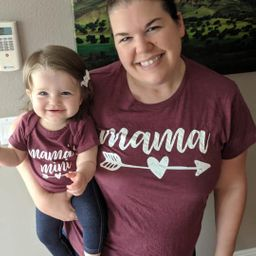Our Discord hit 10K members! 🎉 Meet students and ask top educators your questions.Join Here!Numerade Educator

# Find the mean, median, and mode.$$81,83,91,97$$

## mean $=88,$ median $=87$ and mode $=$ does not exist

#### Topics

No Related Subtopics

### Discussion

You must be signed in to discuss.

### Video Transcript

in order to find the me and that walked it had all of these numbers up and divide by the number of items in our list. When we add 81 83 91 97 we get 3 52 divided by four, the number of items in the list, and that equals out to 88. Next, we'll find the motor, the motives, the number that occurs the most number of times. And you could see that every number in this list occurs just once, so there is no mode finally to find the medium will take the middle number. Since we have this in numerical order and there are only four members in our list will take the two middle. We'll add those together 83 91 then we'll divide by two. And when we do that, we get 87Other Schools

#### Topics

No Related Subtopics